# R S Aggarwal Solutions for Class 10 Maths Chapter 13 Trigonometric Identities Exercise 13A

R S Aggarwal Maths Solutions for Chapter 13 exercise 13A is equipped with all the questions and answers provided in the textbook. You can download the pdf by clicking on the link given below. This exercise helps students in solving problems based on various trigonometric identities. Download R S Aggarwal Solutions of Class 10 and score well in your examinations.

## Download PDF of R S Aggarwal Solutions for Class 10 Maths Chapter 13 Trigonometric Identities Exercise 13A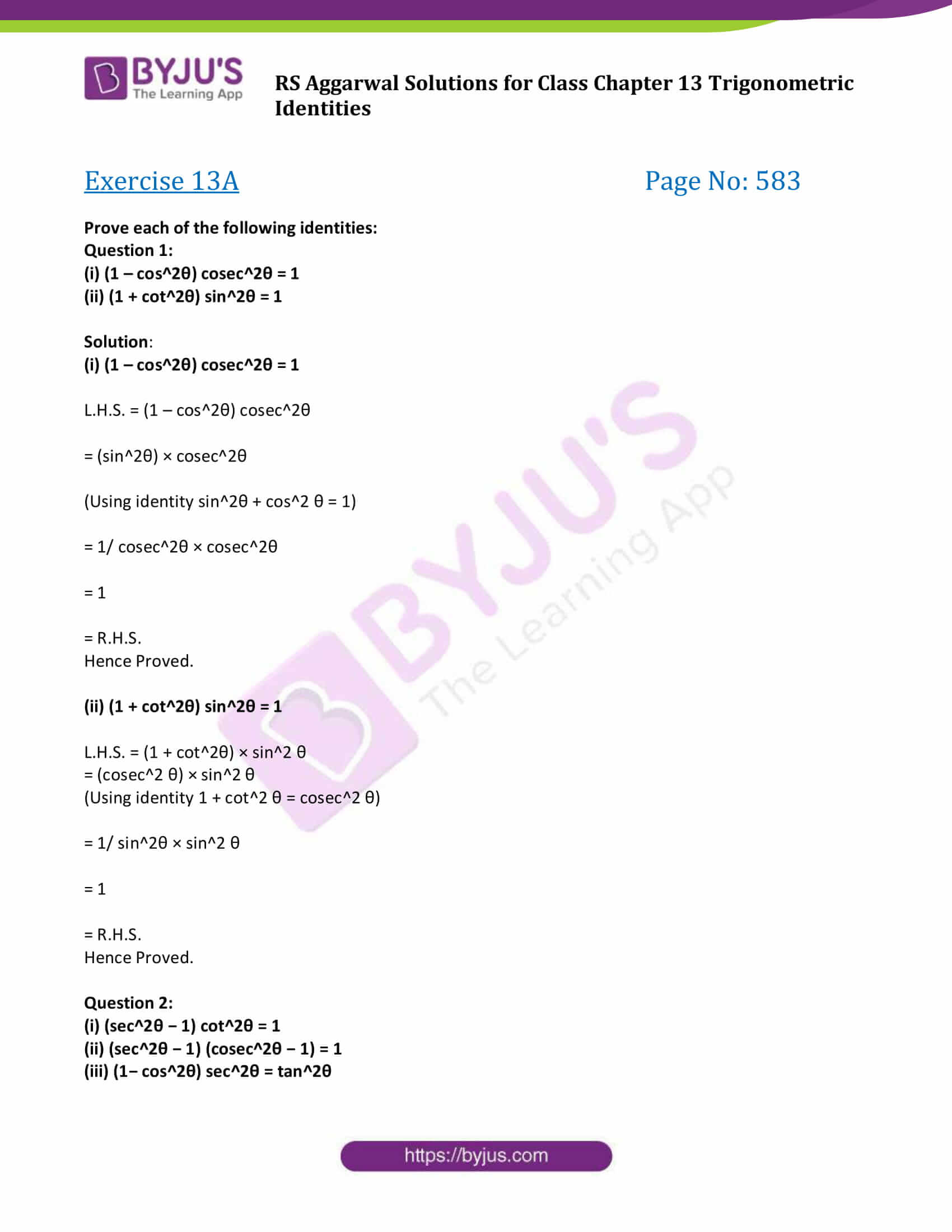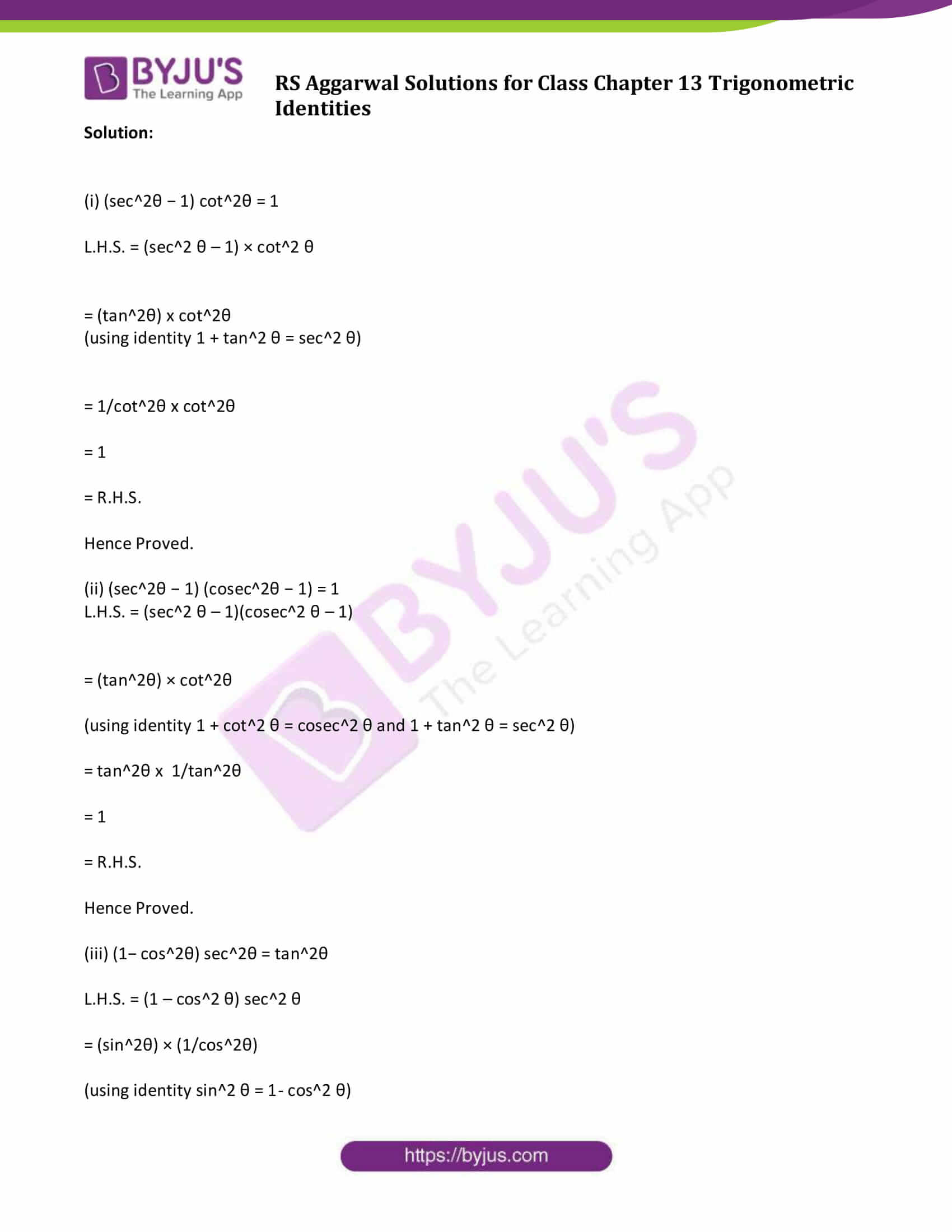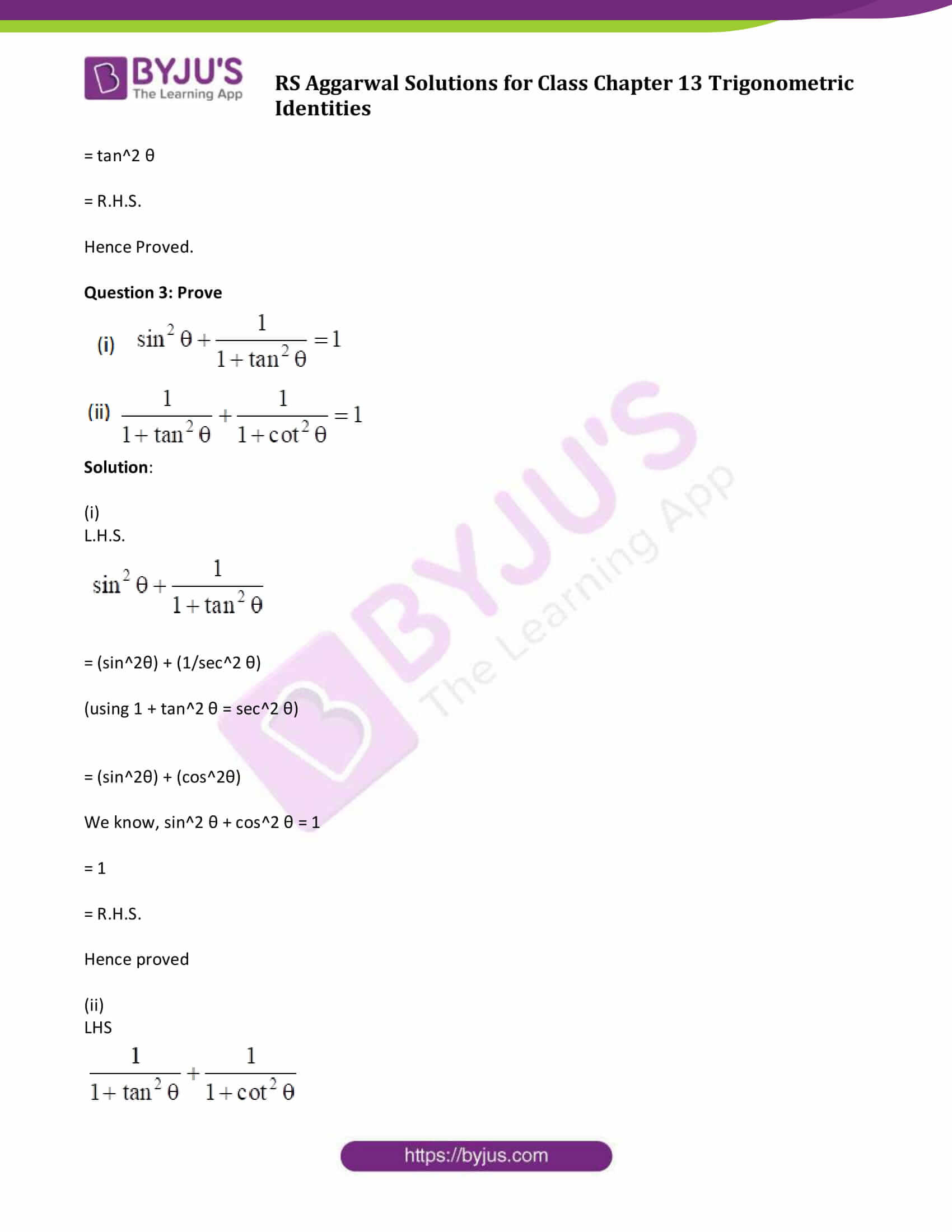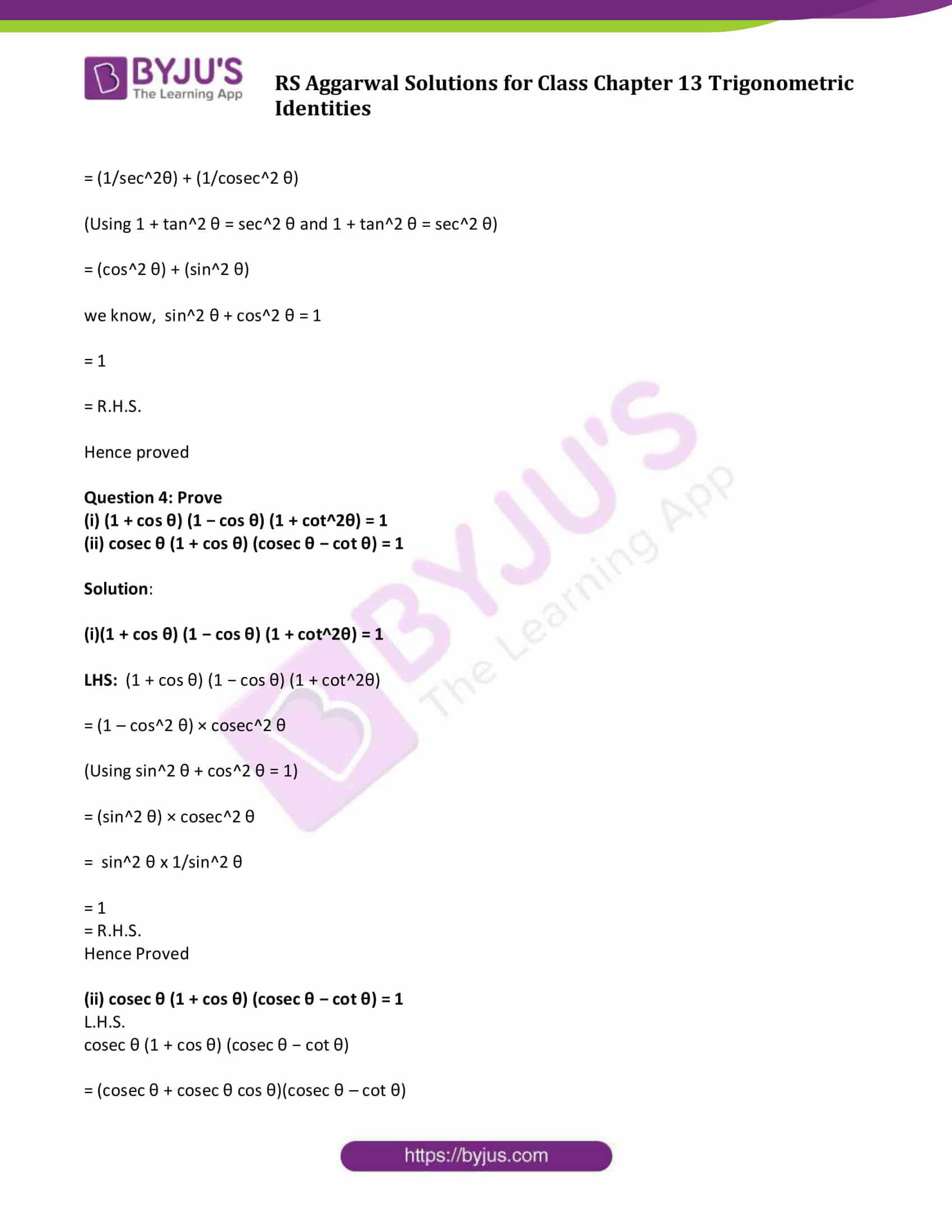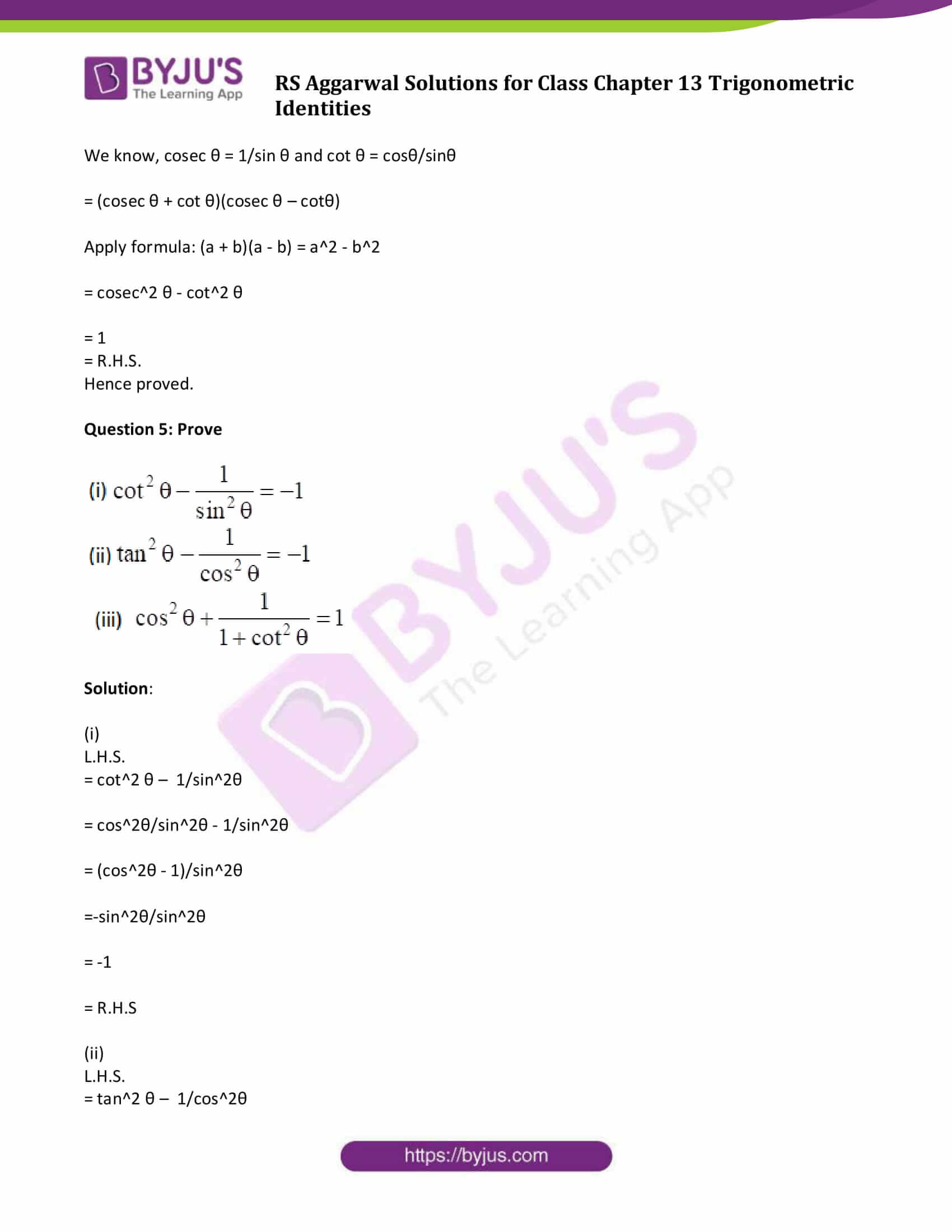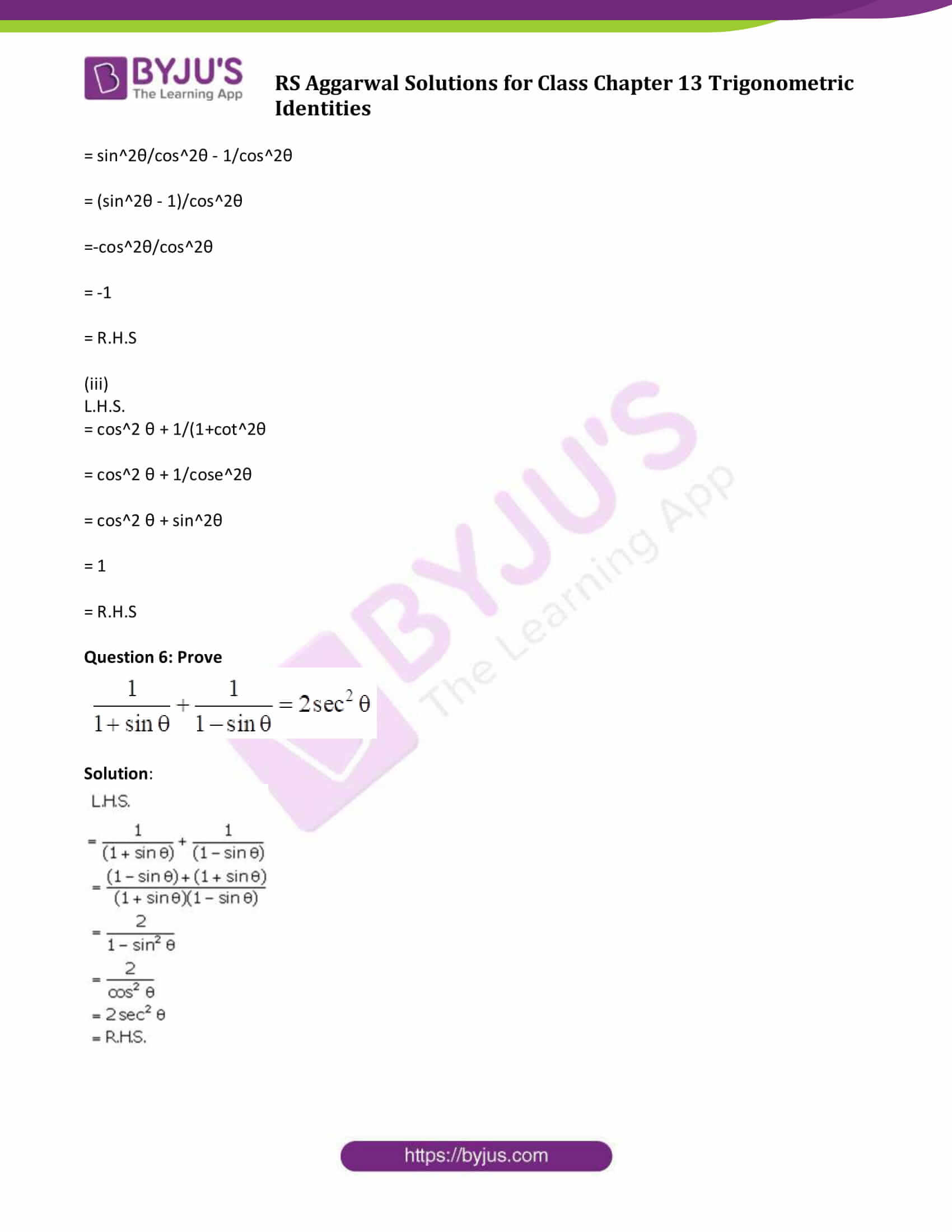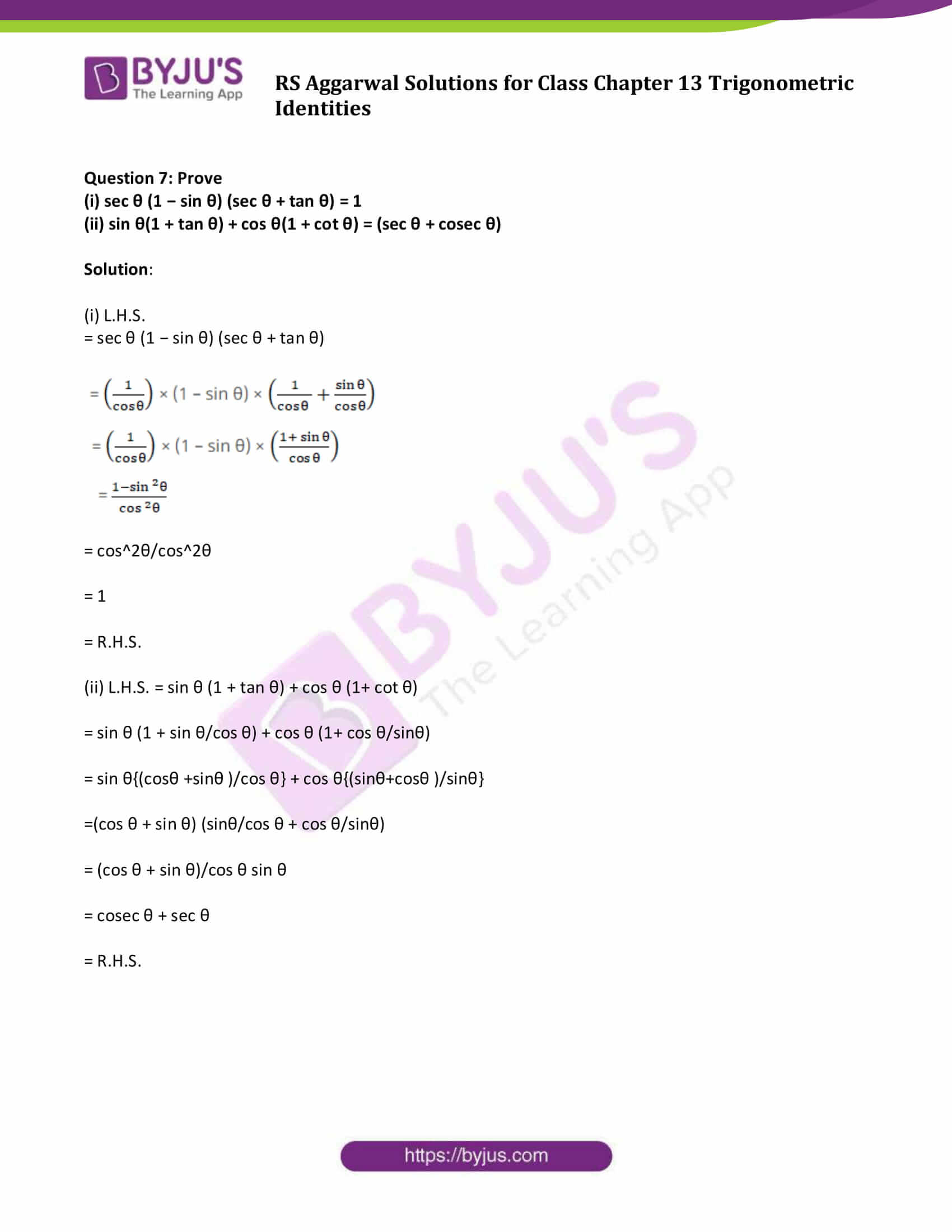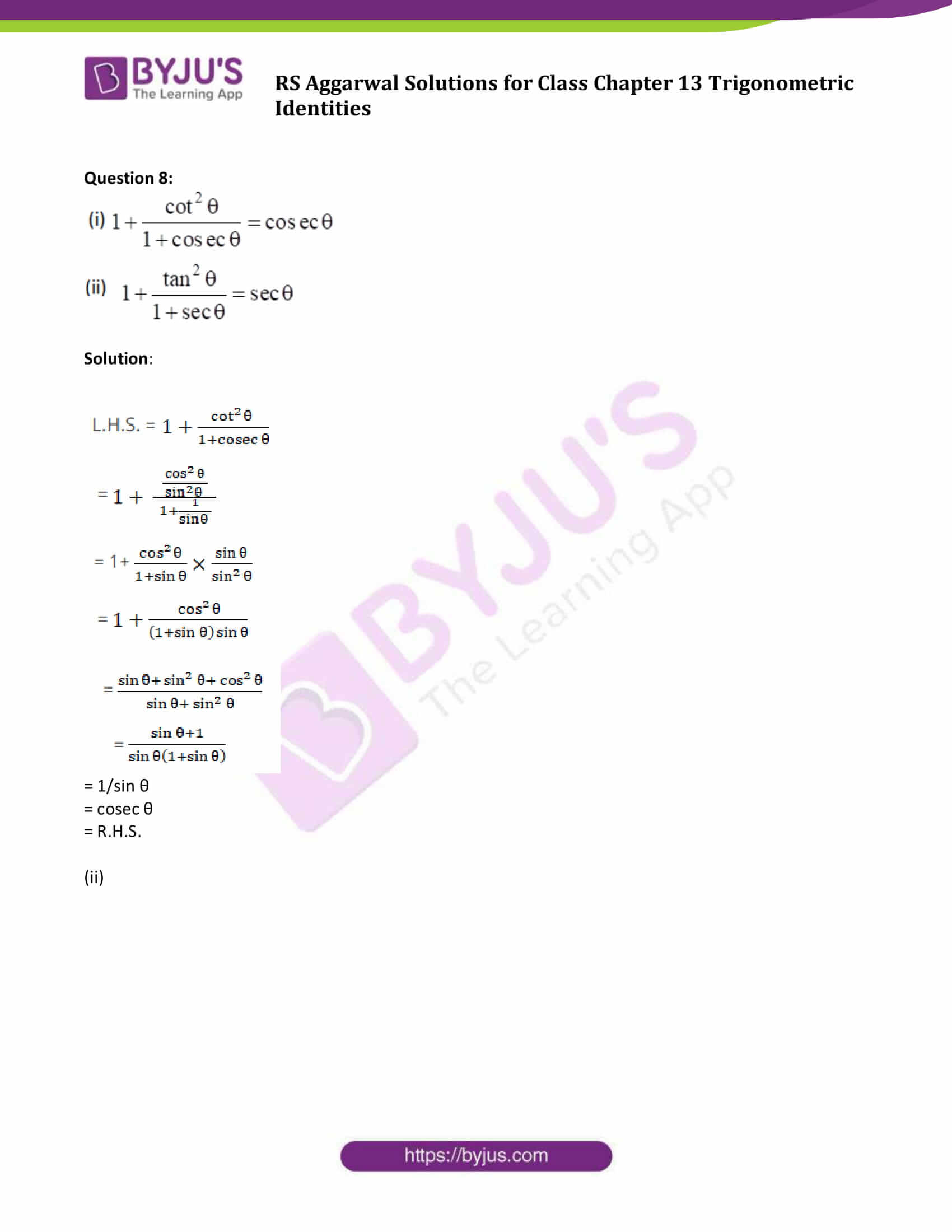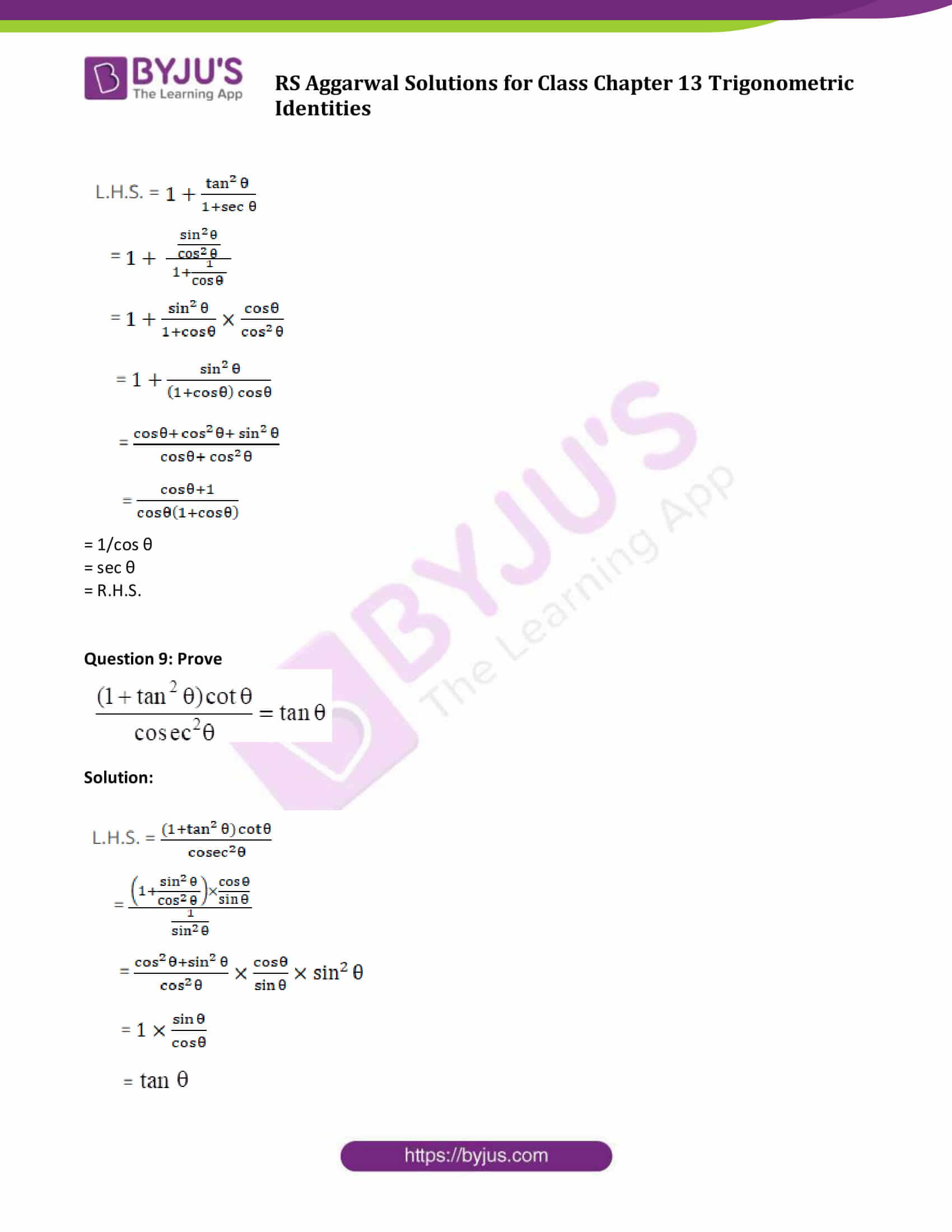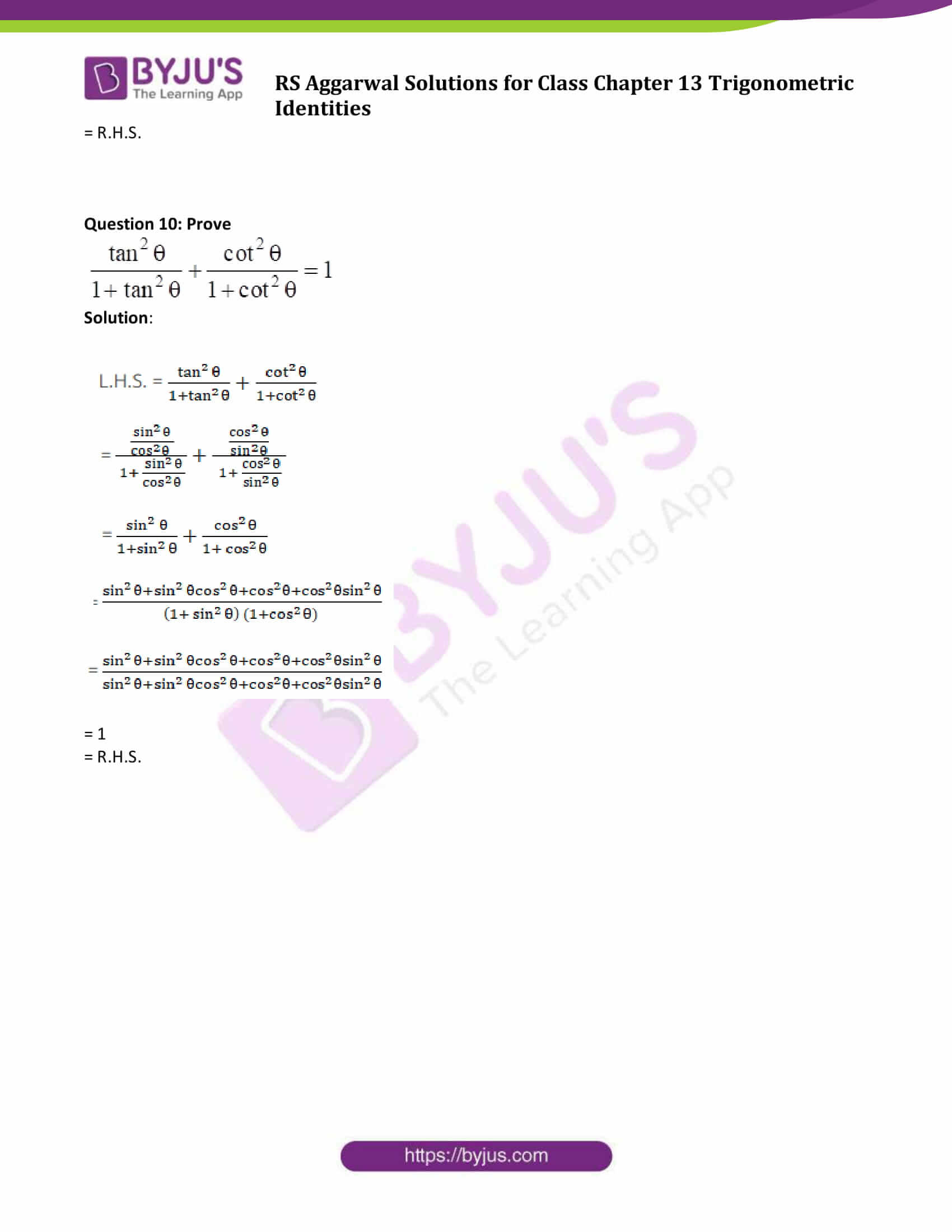### Access other exercise solutions of Class 10 Maths Chapter 13 Trigonometric Identities

Exercise 13 B Solutions

Exercise 13 C Solutions

### Access Solutions to Maths R S Aggarwal Class 10 Chapter 13 Trigonometric Identities Exercise 13A

Prove each of the following identities:

Question 1:

(i) (1 – cos2θ) cosec2θ = 1

(ii) (1 + cot2θ) sin2θ = 1

Solution:

(i) (1 – cos2θ) cosec2θ = 1

L.H.S. = (1 – cos2θ) cosec2θ

= (sin2θ) × cosec2θ

(Using identity sin2θ + cos2 θ = 1)

= 1/ cosec2θ × cosec2θ

= 1

= R.H.S.

Hence Proved.

(ii) (1 + cot2θ) sin2θ = 1

L.H.S. = (1 + cot2θ) × sin2 θ

= (cosec2 θ) × sin2 θ

(Using identity 1 + cot2 θ = cosec2 θ)

= 1/ sin2θ × sin2 θ

= 1

= R.H.S.

Hence Proved.

Question 2:

(i) (sec2θ − 1) cot2θ = 1

(ii) (sec2θ − 1) (cosec2θ − 1) = 1

(iii) (1− cos2θ) sec2θ = tan2θ

Solution:

(i) (sec2θ − 1) cot2θ = 1

L.H.S. = (sec2 θ – 1) × cot2 θ

= (tan2θ) x cot2θ

(using identity 1 + tan2 θ = sec2 θ)

= 1/cot2θ x cot2θ

= 1

= R.H.S.

Hence Proved.

(ii) (sec2θ − 1) (cosec2θ − 1) = 1

L.H.S. = (sec2 θ – 1)(cosec2 θ – 1)

= (tan2θ) × cot2θ

(using identity 1 + cot2 θ = cosec2 θ and 1 + tan2 θ = sec2 θ)

= tan2θ x 1/tan2θ

= 1

= R.H.S.

Hence Proved.

(iii) (1− cos2θ) sec2θ = tan2θ

L.H.S. = (1 – cos2 θ) sec2 θ

= (sin2θ) × (1/cos2θ)

(using identity sin2 θ = 1- cos2 θ)

= tan2 θ

= R.H.S.

Hence Proved.

Question 3: Prove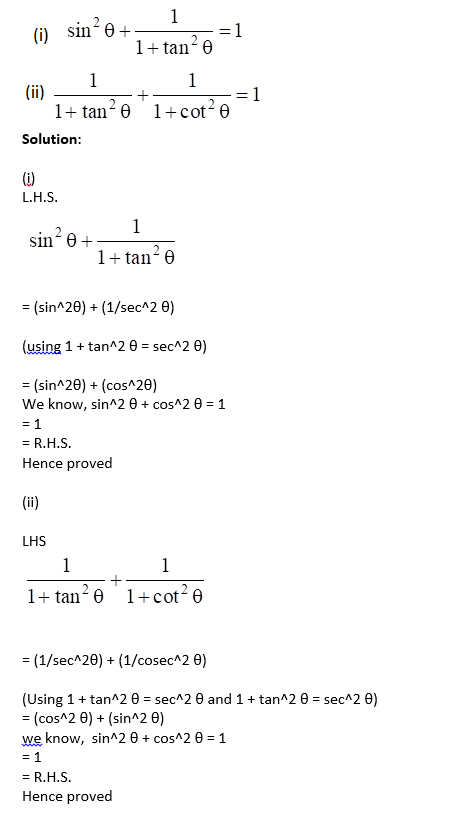Question 4: Prove

(i) (1 + cos θ) (1 − cos θ) (1 + cot2θ) = 1

(ii) cosec θ (1 + cos θ) (cosec θ − cot θ) = 1

Solution:

(i)(1 + cos θ) (1 − cos θ) (1 + cot2θ) = 1

LHS: (1 + cos θ) (1 − cos θ) (1 + cot2θ)

= (1 – cos2 θ) × cosec2 θ

(Using sin2 θ + cos2 θ = 1)

= (sin2 θ) × cosec2 θ

= sin2 θ x 1/sin2 θ

= 1

= R.H.S.

Hence Proved

(ii) cosec θ (1 + cos θ) (cosec θ − cot θ) = 1

L.H.S.

cosec θ (1 + cos θ) (cosec θ − cot θ)

= (cosec θ + cosec θ cos θ)(cosec θ – cot θ)

We know, cosec θ = 1/sin θ and cot θ = cosθ/sinθ

= (cosec θ + cot θ)(cosec θ – cotθ)

Apply formula: (a + b)(a – b) = a2 – b2

= cosec2 θ – cot2 θ

= 1

= R.H.S.

Hence proved.

Question 5: Prove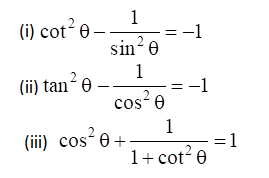Solution:

(i)

L.H.S.

= cot2 θ – 1/sin2θ

= cos2θ/sin2θ – 1/sin2θ

= (cos2θ – 1)/sin2θ

=-sin2θ/sin2θ

= -1

= R.H.S

(ii)

L.H.S.

= tan2 θ – 1/cos2θ

= sin2θ/cos2θ – 1/cos2θ

= (sin2θ – 1)/cos2θ

=-cos2θ/cos2θ

= -1

= R.H.S

(iii)

L.H.S.

= cos2 θ + 1/(1+cot2θ

= cos2 θ + 1/cose2θ

= cos2 θ + sin2θ

= 1

= R.H.S

Question 6: Prove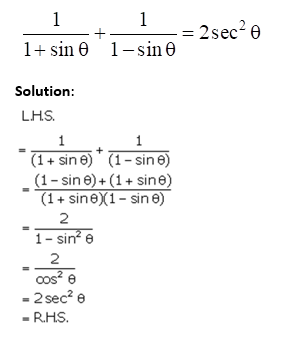Question 7: Prove

(i) sec θ (1 − sin θ) (sec θ + tan θ) = 1

(ii) sin θ(1 + tan θ) + cos θ(1 + cot θ) = (sec θ + cosec θ)

Solution:

(i) L.H.S.

= sec θ (1 − sin θ) (sec θ + tan θ)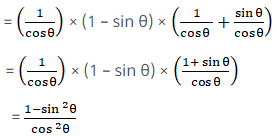= cos2θ/cos2θ

= 1

= R.H.S.

(ii) L.H.S. = sin θ (1 + tan θ) + cos θ (1+ cot θ)

= sin θ (1 + sin θ/cos θ) + cos θ (1+ cos θ/sinθ)

= sin θ{(cosθ +sinθ )/cos θ} + cos θ{(sinθ+cosθ )/sinθ}

=(cos θ + sin θ) (sinθ/cos θ + cos θ/sinθ)

= (cos θ + sin θ)/cos θ sin θ

= cosec θ + sec θ

= R.H.S.

Question 8: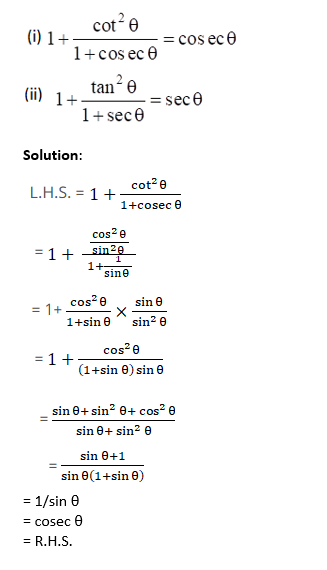(ii)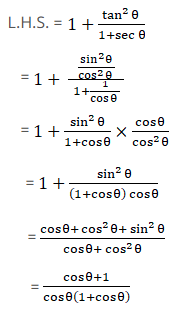= 1/cos θ

= sec θ

= R.H.S.

Question 9: Prove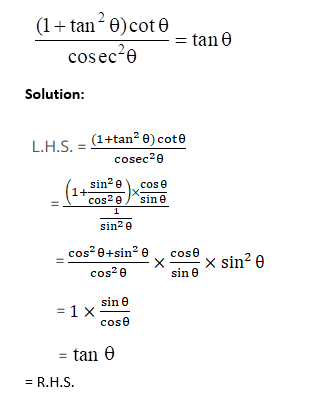Question 10: Prove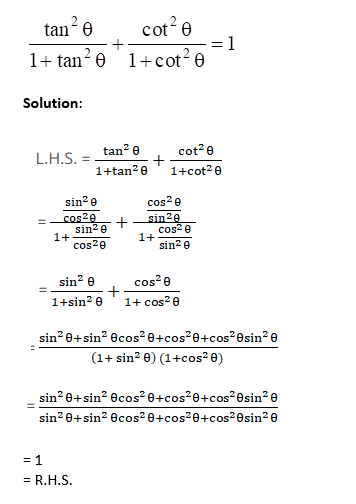## R S Aggarwal Solutions for Class 10 Maths Chapter 13 Trigonometric Identities Exercise 13A

Class 10 Maths Chapter 13 Trigonometric Identities Exercise 13A is based on the following identities:

• sin2 θ = 1 − cos2 θ
• cos2 θ = 1 − sin2 θ
• tan2 θ + 1 = sec2 θ
• tan2 θ = sec2 θ − 1
• cot2 θ + 1 = csc2 θ
• cot2 θ = csc2 θ − 1